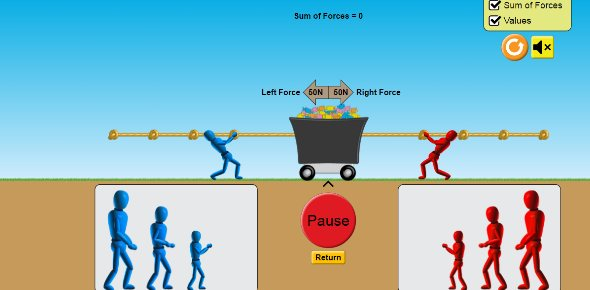# Force And Motion

6 Questions | Total Attempts: 69Settings.

Related Topics
• 1.
In what unit do we measure force?
• A.

Meters

• B.

Grams

• C.

Newtons

• D.

Liters

• 2.
What type of equipment is used to measure force?
• A.

Balance

• B.

• C.

Ruler

• D.

Spring scale

• 3.
What can you tell about the forces from the arrows a below?
• A.

The forces are unbalanced.

• B.

The forces are balanced.

• C.

The force to the left is larger.

• D.

The force from the right is larger.

• 4.
Based on the forces acting on the ball, will the motion of the ball change and in what direction?
• A.

No, the motion of the ball with not change

• B.

Yes, the ball will move to the right

• C.

Yes, the ball will move to the left

• D.

Yes, the ball will move up

• 5.
In which direction is the car moving?
• A.

The car will move forward

• B.

The car will move backward

• C.

The car will not move

• D.

Option 4

• 6.
Are the forces on the car balanced or unbalanced?
• A.

Balanced

• B.

Unbalanced

• C.

Option 3

• D.

Option 4